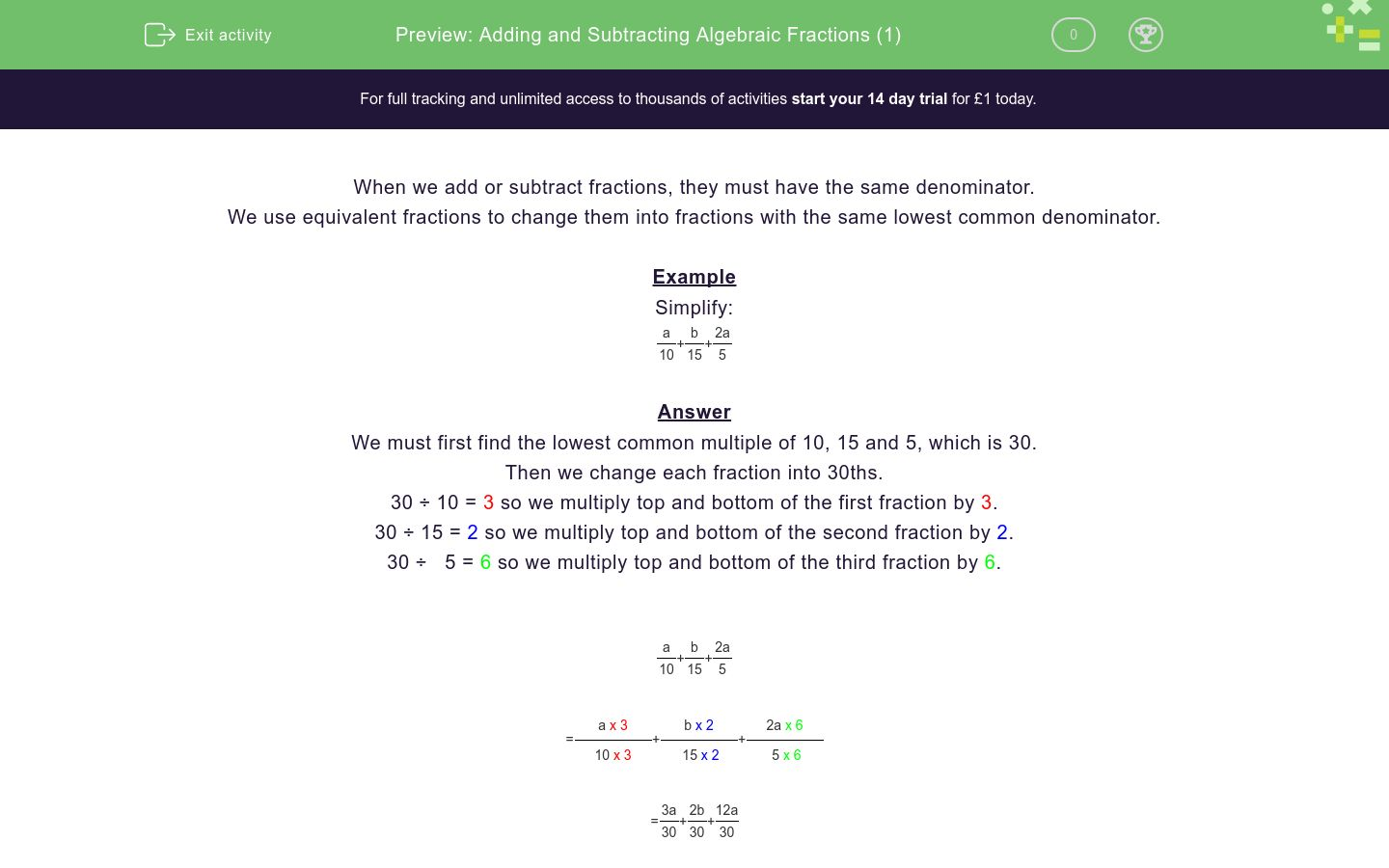# Adding and Subtracting Algebraic Fractions (1)

In this worksheet, students add or subtract algebraic fractions giving their answer as a single fraction.Key stage:  KS 4

Curriculum topic:  Algebra

Curriculum subtopic:  Simplify/Manipulate Algebraic Expressions/Fractions

Difficulty level:\$appendString = '&new=1'; \$_SESSION['guest_aId'] = rand(); \$urlToRedirect = FRAMEWORK_PATH . 'preview/worksheet/introduction?aId=' . \$_SESSION['guest_aId'] . '&wId=' . \$wsData['id'] . '&usertype=guest' . \$appendString; ?>

### QUESTION 1 of 10

When we add or subtract fractions, they must have the same denominator.

We use equivalent fractions to change them into fractions with the same lowest common denominator.

Example

Simplify:

 a + b + 2a 10 15 5

Answer

We must first find the lowest common multiple of 10, 15 and 5, which is 30.

Then we change each fraction into 30ths.

30 ÷ 10 = 3 so we multiply top and bottom of the first fraction by 3.

30 ÷ 15 = 2 so we multiply top and bottom of the second fraction by 2.

30 ÷   5 = 6 so we multiply top and bottom of the third fraction by 6.

 a + b + 2a 10 15 5

 = a x 3 + b x 2 + 2a x 6 10 x 3 15 x 2 5 x 6

 = 3a + 2b + 12a 30 30 30

 = 15a + 2b 30

Simplify the following and select the correct answer:

 a + a + a 10 15 5

Answers

 1 a 2. 9a 3. 11a 10 30 30

1.

2.

3.

Simplify the following and select the correct answer:

 a + b + a 10 15 5

Answers

 1 a + b 2. 9a + 2b 3. a + 2b 15 30 10

1.

2.

3.

Simplify the following and select the correct answer:

 a + b - a 10 15 5

Answers

 1 2b - 3a 2. 9a + 2b 3. a + 2b 30 30 10

1.

2.

3.

Simplify the following and select the correct answer:

 b + b - a 10 15 5

Answers

 1 5b - 6a 2. 9a + 2b 3. 2b - a 30 30 20

1.

2.

3.

Simplify the following and select the correct answer:

 b + b - b 10 15 5

Answers

 1 11b 2. b 3. -b 30 30 30

1.

2.

3.

Simplify the following and select the correct answer:

 b + b + b 10 15 5

Answers

 1 11b 2. b 3. -b 30 30 30

1.

2.

3.

Simplify the following and select the correct answer:

 b + b + b 6 9 12

Answers

 1 11b 2. 13b 3. b 30 36 9

1.

2.

3.

Simplify the following and select the correct answer:

 a + b + b 6 9 12

Answers

 1 abb 2. 6a + 7b 3. a + 2b 36 36 9

1.

2.

3.

Simplify the following and select the correct answer:

 a + 2b + b 6 9 18

Answers

 1 a + 3b 2. 3a + b 3. 3a + 5b 36 36 18

1.

2.

3.

Simplify the following and select the correct answer:

 5a + 2b + b 6 4 18

Answers

 1 5a + 3b 2. 15a + 10b 3. 3a + 5b 36 18 18

1.

2.

3.

• Question 1

Simplify the following and select the correct answer:

 a + a + a 10 15 5

Answers

 1 a 2. 9a 3. 11a 10 30 30

CORRECT ANSWER
3.
• Question 2

Simplify the following and select the correct answer:

 a + b + a 10 15 5

Answers

 1 a + b 2. 9a + 2b 3. a + 2b 15 30 10

CORRECT ANSWER
2.
• Question 3

Simplify the following and select the correct answer:

 a + b - a 10 15 5

Answers

 1 2b - 3a 2. 9a + 2b 3. a + 2b 30 30 10

CORRECT ANSWER
1.
• Question 4

Simplify the following and select the correct answer:

 b + b - a 10 15 5

Answers

 1 5b - 6a 2. 9a + 2b 3. 2b - a 30 30 20

CORRECT ANSWER
1.
• Question 5

Simplify the following and select the correct answer:

 b + b - b 10 15 5

Answers

 1 11b 2. b 3. -b 30 30 30

CORRECT ANSWER
3.
• Question 6

Simplify the following and select the correct answer:

 b + b + b 10 15 5

Answers

 1 11b 2. b 3. -b 30 30 30

CORRECT ANSWER
1.
• Question 7

Simplify the following and select the correct answer:

 b + b + b 6 9 12

Answers

 1 11b 2. 13b 3. b 30 36 9

CORRECT ANSWER
2.
• Question 8

Simplify the following and select the correct answer:

 a + b + b 6 9 12

Answers

 1 abb 2. 6a + 7b 3. a + 2b 36 36 9

CORRECT ANSWER
2.
• Question 9

Simplify the following and select the correct answer:

 a + 2b + b 6 9 18

Answers

 1 a + 3b 2. 3a + b 3. 3a + 5b 36 36 18

CORRECT ANSWER
3.
• Question 10

Simplify the following and select the correct answer:

 5a + 2b + b 6 4 18

Answers

 1 5a + 3b 2. 15a + 10b 3. 3a + 5b 36 18 18

CORRECT ANSWER
2.
---- OR ----

Sign up for a £1 trial so you can track and measure your child's progress on this activity.

### What is EdPlace?

We're your National Curriculum aligned online education content provider helping each child succeed in English, maths and science from year 1 to GCSE. With an EdPlace account you’ll be able to track and measure progress, helping each child achieve their best. We build confidence and attainment by personalising each child’s learning at a level that suits them.

Get startedStart your £1 trial today.
Subscribe from £10/month.